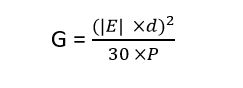# Required Antenna Gain for Desired Field Strength

This online calculator can be used to calculate the required antenna gain using the amplifier power, desired field strength, and the distance from the antenna.
• V/m
• W
• m

### Result

• Antenna Gain
dBi

Required Antenna Gain for Desired Field Strength

Antenna gain is a measure of the maximum effectiveness with which the antenna can radiate the power delivered to it by the transmitter towards a target. Antenna gain is typically given the symbol G.

This calculator will determine the antenna gain when the desired field strength, distance, and amplifier power are entered into the input.

Antenna gain is given by the formula:

WhereG is the antenna gain in dBi

E is the desired field strength in V/m

D is the distance in m

P is the amplifier power in W